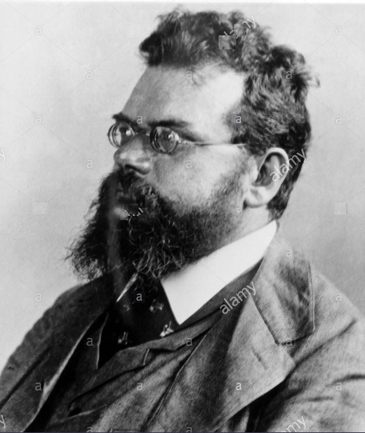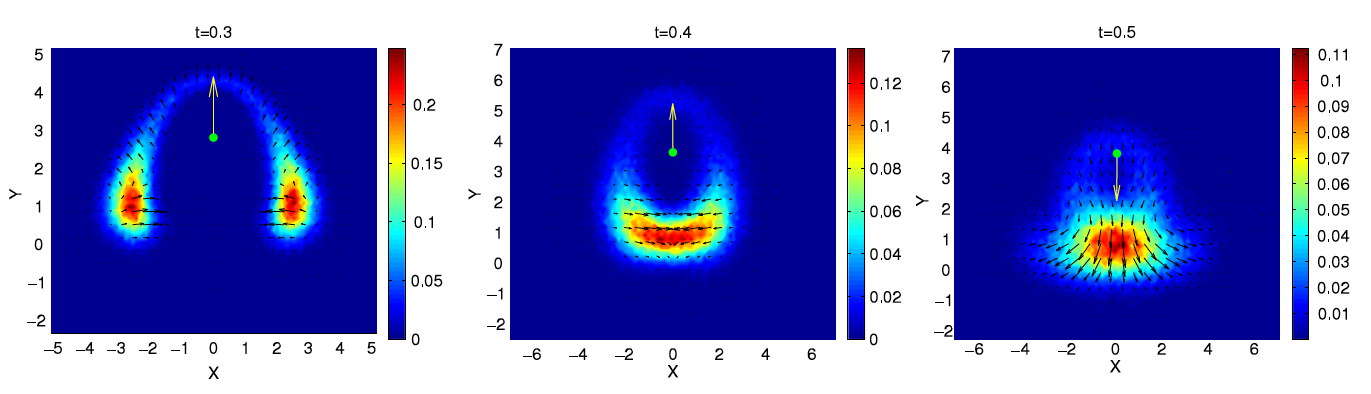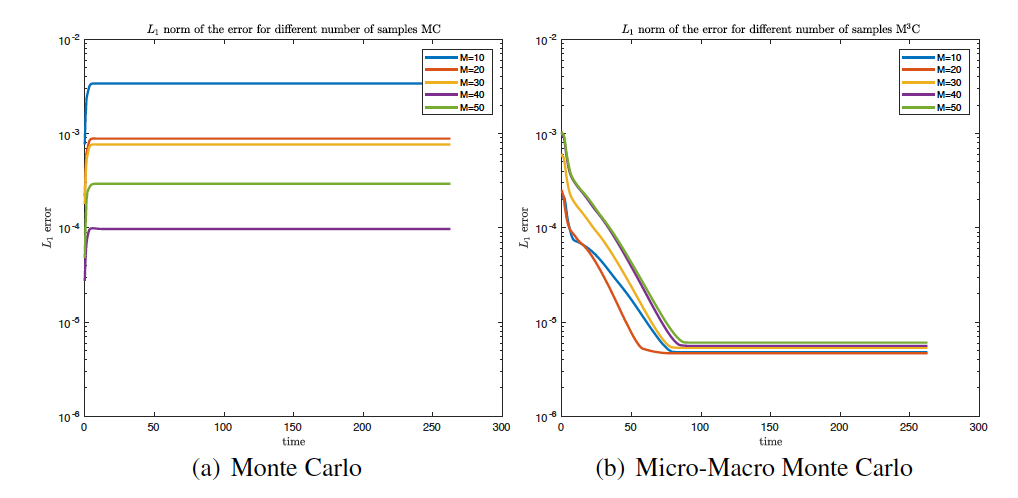# Uncertainty quantification and the Boltzmann legacy

After the celebrated Boltzmann equation in 1872, which describes the time evolution of a rarefied gas, kinetic equations have been applied to model a variety of phenomena whose multiscale nature cannot be described by a standard macroscopic approach.

The equation arises not by analyzing the individual positions and velocity of each particle in the fluid but by considering a probability distribution f(x,v,t) for the position and velocity of a typical particle—that is, the probability that the particle occupies a given very small region of space centered at the position x, and has velocity nearly equal to v (thus occupying a very small region of velocity space), at an instant of time t.Ludwig Boltzmann (1844-1906)

Recently, kinetic equations have found novel applications in the study of emergent behaviors in complex systems characterized by the spontaneous formation of spatio-temporal structures as a result of simple local interactions between agents. These include the study of birds’ flocks, insects’ swarming, crowd dynamics, opinions’ formation, stock markets and wealth distributions. Clearly, the basic entities in these fields differ from physical particles in that they already have an intermediate complexity themselves and are commonly denoted as agents (see ).Milling behavior in fishes (left) and flocking of starlings (right)

One of the major difficulties in applying the classical toolbox of kinetic theory to these new fields is the lack of fundamental principles which define the microscopic dynamic. A degree of uncertainty is therefore implicitly embedded in such models, since most modeling parameters can be assigned only as statistical information from experimental results (see  for recent surveys).

In spite of the vast amount of existing research, both theoretically and numerically (see [3,8]), the study of kinetic equations has mostly remained deterministic and ignored uncertainty. In reality, there are many sources of uncertainties that can arise in these equations:

• Incomplete knowledge of the interaction mechanism between particles/agents.

• Imprecise measurements of the initial and boundary data.

• Other sources of uncertainty like forcing and geometry, etc.

Understanding the impact of these uncertainties is critical to the simulations of the complex kinetic systems to validate the kinetic models, and will allow scientists and engineers to obtain more reliable predictions and perform better risk assessment.Simulation of a flock attacked by a predator using the method developed in 

The development of numerical methods for kinetic equations presents several difficulties due to the high dimensionality and the intrinsic structural properties of the solution. Non-negativity of the distribution function, conservation of invariant quantities, entropy dissipation and steady states are essential in order to compute qualitatively correct solutions. Preservation of these structural properties is even more challenging in presence of uncertainties which contribute to curse of dimensionality.

Uncertainty quantification (UQ) in kinetic equations represents a computational challenge for many reasons. Simple UQ tasks such as the estimation of statistical properties of the solution typically require multiple calls to a deterministic solver. A single solver call is already very expensive for such complex mathematical models.Convergence acceleration of Micro-Macro Monte Carlo versus standard Monte Carlo

Researchers at DMCS have a recognized international experience in the numerical analysis of kinetic equations with fundamental contributions in the development of fast spectral methods, Monte Carlo methods and asymptotic preserving schemes (see the recent review in  for example).  Recently they developed novel approaches to UQ of kinetic equations based on generalized Polynomial Chaos expansions at a particle level in order to reduce the problem dimension and maintain the main physical properties of the solution (see ) and on micro-macro Monte Carlo techniques which using control variate estimators based on the local equilibrium are capable to accelerate the slow statistical convergence of Monte Carlo methods (see [4, Chapter 5]).

References

1. Albi G., Pareschi L. (2013), Binary interaction algorithms for the simulation of flocking and swarming dynamics. Multiscale Modeling & Simulation 11, 1-29.

2. Carrillo J.A., Pareschi L., Zanella M. (2017), Particle gPC methods for mean field models of swarming with uncertainties, arXiv:1712.01677.

3. Dimarco G., Pareschi L. (2015), Numerical methods for kinetic equations. Acta Numerica.

4. Jin S., Pareschi L., eds (2018), Uncertainty quantification in hyperbolic and kinetic equations. SEMA-SIMAI Series in Applied Mathematics, Springer.

5. Mouhot C., Pareschi L. (2006), Fast algorithms for computing the Boltzmann collision operator. Mathematics of Computation 75, 1833-1852.

6. Pareschi L., Toscani G. (2013), Interacting multi-agents systems: kinetic equations and Monte Carlo methods. Oxford University Press, (2013)

7. Pareschi L., Toscani G., Villani C. (2003), Spectral methods for the non cut-off Boltzmann equation and numerical grazing collision limit. Numerische Mathematik 93, 527-548.

8. Villani C. (2002), A survey of mathematical topics in kinetic theory. Handbook of fluid mechanics, S. Friedlander and D. Serre, Eds. Elsevier Publ. North-Holland vol. I, 71-305.

Contact

Prof. Lorenzo Pareschi

Department of Mathematics and Computer Science

University of Ferrara

Via Machiavelli 30, 44121 Ferrara

e-mail: lorenzo.pareschi@unife.it

Filed under: